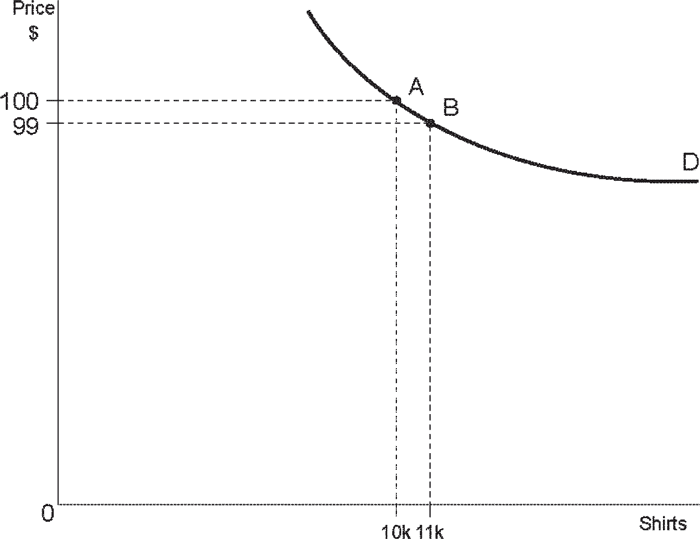# Elasticity

## Understanding What is Elasticity

The term elasticity in economics refers to the extent to which data of any kind are affected by changes in another factor. For example: how is the quantity of shirts bought affected by changes in the price of shirts.

The elasticity is expressed as a number. The higher the number, the greater the degree of elasticity.

The exact definition of elasticity for a particular product:

Elasticity = % change in quantity

Change of 1% in price

Example

We are examining the degree of elasticity of demand for shirts, which is the change (percentage) in the quantity of shirts that we want to buy as a result of a change of 1% in the price of shirts. In our example the initial stage is that the price of shirts is \$100 and the quantity demanded is 10k shirts (Graph 14.1). (k = 1,000, 10k = 10,000).

The price of shirts is reduced to \$99 (= a reduction in price of 1%).

The quantity demanded increases to 11k, an increase of 10% (point B)

In this example, the elasticity at Point A is 10.

Remember: The elasticity is not uniform over the demand curve. Thus it is important when discussing elasticity to state the point for which calculation is being made, in the following manner:

The elasticity at point A is 10. The elasticity at point B is 9 (assuming this is the case)

Graph 14.1